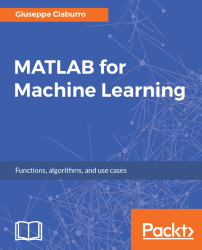•#### MATLAB for Machine Learning#### Overview of this book

MATLAB is the language of choice for many researchers and mathematics experts for machine learning. This book will help you build a foundation in machine learning using MATLAB for beginners. You’ll start by getting your system ready with t he MATLAB environment for machine learning and you’ll see how to easily interact with the Matlab workspace. We’ll then move on to data cleansing, mining and analyzing various data types in machine learning and you’ll see how to display data values on a plot. Next, you’ll get to know about the different types of regression techniques and how to apply them to your data using the MATLAB functions. You’ll understand the basic concepts of neural networks and perform data fitting, pattern recognition, and clustering analysis. Finally, you’ll explore feature selection and extraction techniques for dimensionality reduction for performance improvement. At the end of the book, you will learn to put it all together into real-world cases covering major machine learning algorithms and be comfortable in performing machine learning with MATLAB.
Title PageCreditsForewordwww.PacktPub.comCustomer FeedbackPrefaceFree Chapter
Getting Started with MATLAB Machine LearningImporting and Organizing Data in MATLABFrom Data to Knowledge DiscoveryFinding Relationships between Variables - Regression TechniquesPattern Recognition through Classification AlgorithmsIdentifying Groups of Data Using Clustering MethodsSimulation of Human Thinking - Artificial Neural NetworksImproving the Performance of the Machine Learning Model - Dimensionality ReductionMachine Learning in Practice## Neural Network Toolbox

The Neural Network Toolbox provides algorithms, pre-trained models, and apps to create, train, visualize, and simulate neural networks with one hidden layer (called shallow neural networks) and neural networks with several hidden layers (called deep neural networks). Through the use of the tools offered, we can perform classification, regression, clustering, dimensionality reduction, time series forecasting, and dynamic system modeling and control.

There are several ways to use the Neural Network Toolbox software; the essential ones are the four ways that are listed here:

• The most comfortable one uses MATLAB graphical user interfaces. We can start the main window through the `nnstart` command. In this way, we can automatically perform the following tasks: function fitting (`nftool`), pattern recognition (`nprtool`), data clustering (`nctool`), time series analysis (`ntstool`).
• We can use basic command-line operations. Command-line operations offer greater flexibility but require...